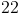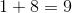HSPT Math : Problem Solving

Example Questions

Example Question #61 : Problem Solving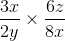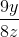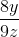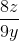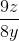Explanation:

Multiplying an algebraic fraction is the same as multiplying fractions. Just multiply the numerators and the denominators and simplify.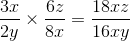To simply, divide the numerator and the denominator by the greatest common factor of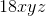and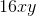, which is.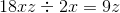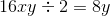Example Question #62 : Problem Solving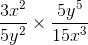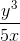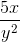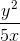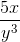Explanation: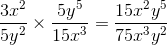To simplify divide the numerator and the denominator by the Greatest Common Factor, which is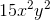.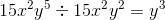(when you are dividing, subtract the exponents)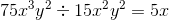Example Question #63 : Problem Solving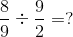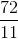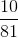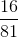Explanation:

To divide fractions, you multiply by the reciprocal (flip the denominator and multiply instead.  \

So the problem now looks like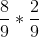.

Then you multiply across,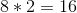and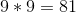..

Example Question #1 : Percentages

The price of a suit after a 20% employee discount is $240.00. What is the original price? Possible Answers: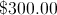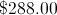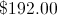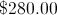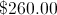Correct answer:Explanation: Deducting a 20% discount is the same as charging 80% of the price, so$240.00 is 80% of the original price, which we call. Just solve this equation: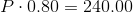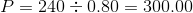The suit originally cost $300.00 Example Question #2 : Percentages Express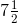% as a fraction in lowest terms. Possible Answers: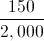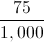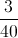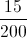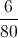Correct answer:Explanation: Put the percent over 100 and simplify as follows: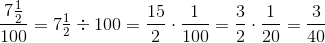Note: all of these choices are equivalent to%; but only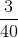is in lowest terms. Example Question #3 : Percentages A store normally sells a certain coat for$40.  For the holidays it offers a sale where the new price of the coat is now $30. What percent discount is the store selling the coat for? Possible Answers: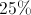discount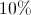discount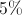discount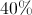discount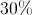discount Correct answer:discount Explanation: The coat is$10 cheaper than normal.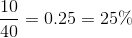Marshal saves 10% of his paycheck each week. If Marshal earned $652.20 this week, approximately how much money did he save? Possible Answers: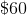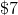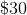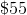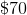Correct answer:Explanation: The word approximately tells you that you need to estimate to get the answer.$652.20 can round up to $700 because 52.20 is more than half of 100. Since 10% is equal to the decimal 0.10, we multiply 700 by this number in order to find out what 10% of 700 is.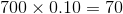The answer isExample Question #1 : Percentage The sales tax rate for a particular locality is 9%. How much will be paid after tax for$154.92 worth of groceries?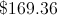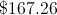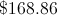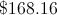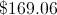Explanation:

Multiply the price of the groceries before tax - $154.92 - by the decimal equivalent of 9% , which is 0.09. Round this tax to the nearest hundredth (cents), then add to the price of the groceries. Tax: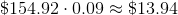Price after tax: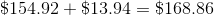Example Question #7 : Percentage Susan has a coupon for 10% off any one item at her favorite department store. There is also a storewide sale of 50% off all purchases. If Susan wants to by a shirt originally priced at$42, how much will she pay?

$20.00$21.00

$16.50$37.80

$18.90 Correct answer:$18.90

Explanation:

The storewide sale discounts the shirt by 50%: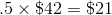Susan also has the 10% off coupon, so she's really paying 90% of the cost of the shirt: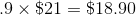Example Question #5 : Percentages

Exactlyof the plants in a garden are tomato plants.  Which of the following could be the total number of plants in the garden?